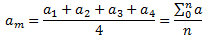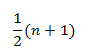Home/All Courses/CBSE/NCERT/NCERT Solutions For Class 9/NCERT Solution For Class 9 Maths/NCERT Solutions for Class 9 Maths Chapter 14 – Statistics

## NCERT Solutions for Class 9 Maths Chapter 14 – Statistics

NCERT solutions for class 9 maths chapter 14 pdf covers the topic of Collection of Data,  Presentation of Data, Graphical Representation of Data. The chapter also discusses about mean, median, mode. To get in-depth information and statistics class 9 notes you can refer to ncert solutions for class 9 maths chapter 14 pdf given below.

## Statistics

Statistics deals with collection, presentation, analysis and interpretation of numerical data.

## Collection of data

The first step in any investigation is collection of data. The data may be collected for the whole population or for a sample only.

## Presentation

1)Arranging data in a order to study their salient features is called presentation of data
2) Data arranged in ascending or descending order is called arrayed data or an array
3) organize numerical information in the form of tables, graphs, and charts.

## Analysis and interpretation

1) Understand statistical techniques underlying decisions that affect our lives and well-being and
make informed decisions.
2) The process by which sense and meaning are made of the data gathered  and applied to clients problem

## More on Presentation of Data

We can divide data into two Forms in terms of Presentation

### Ungrouped data

• The data obtained in original form is called  raw data or ungrouped data
• A frequency distribution table that shows the frequency of each individual value in the given data is called an ungrouped frequency distribution table.

### Grouped data

• The ungrouped data approach becomes very hard with large values of number. So we make groups of suitable size to put the data in a more condensed form and mention the frequency of each group. Such a table is called a grouped frequency distribution table.

## Measures of Central Tendency

Let’s discuss mean median mode:

Mean
The mean value of a variable is defined as the sum of all the values of the variable divided by the number of values.Median
The median of a set of data values is the middle value of the data set when it has been arranged in ascending order.  That is, from the smallest value to the highest value
Median is calculated asWhere n is the number of values in the data
If the number of values in the data set is even, then the median is the average of the two middle values.
Mode
Mode of a statistical data is the value of that variable which has the maximum frequency.

2018-10-31T12:00:51+00:00 Categories: NCERT Solution For Class 9 Maths||0 Comments
Hello